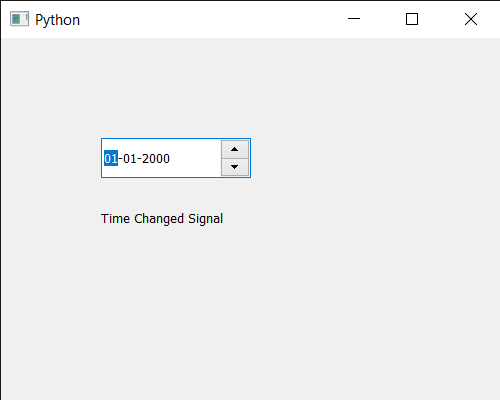Related Articles
PyQt5 QDateEdit – Time Changed Signal
• Last Updated : 07 Jul, 2020

In this article we will see how we can get time changed signal of the QDateEdit. Unlike normal like date we can set date time to it with the help of `setDateTime` method. But also we can set only time as well without changing the date with the help of `setTime` method. This signal is emitted whenever the time of date edit is changed.

In order to do this we use `timeChanged` method with the QDateEdit object

Syntax : date.timeChanged.connect(method)

Argument : It takes method as argument

Return : It returns None

Below is the implementation

 `# importing libraries``from` `PyQt5.QtWidgets ``import` `*` `from` `PyQt5 ``import` `QtCore, QtGui``from` `PyQt5.QtGui ``import` `*` `from` `PyQt5.QtCore ``import` `*` `import` `sys`` ` ` ` `class` `Window(QMainWindow):`` ` `    ``def` `__init__(``self``):``        ``super``().__init__()`` ` `        ``# setting title``        ``self``.setWindowTitle(``"Python "``)`` ` `        ``# setting geometry``        ``self``.setGeometry(``100``, ``100``, ``500``, ``400``)`` ` `        ``# calling method``        ``self``.UiComponents()`` ` `        ``# showing all the widgets``        ``self``.show()`` ` `    ``# method for components``    ``def` `UiComponents(``self``):`` ` `        ``# creating a QDateEdit widget``        ``date ``=` `QDateEdit(``self``)`` ` `        ``# setting geometry of the date edit``        ``date.setGeometry(``100``, ``100``, ``150``, ``40``)`` ` `        ``# creating a label``        ``label ``=` `QLabel(``"GeeksforGeeks"``, ``self``)`` ` `        ``# setting geometry``        ``label.setGeometry(``100``, ``150``, ``200``, ``60``)`` ` `        ``# making label multiline``        ``label.setWordWrap(``True``)`` ` ` ` ` ` `        ``# time change signal``        ``date.timeChanged.connect(``lambda``: method())`` ` `        ``# method called when signal emitted``        ``def` `method():`` ` `            ``# setting text to the label``            ``label.setText(``"Time Changed Signal"``)`` ` ` ` `        ``# date time``        ``time ``=` `QTime(``11``, ``20``, ``0``)`` ` `        ``# setting time``        ``date.setTime(time)``         ` ` ` ` ` ` ` ` ` `# create pyqt5 app``App ``=` `QApplication(sys.argv)`` ` `# create the instance of our Window``window ``=` `Window()`` ` `# start the app``sys.exit(App.``exec``())`

Output :Attention geek! Strengthen your foundations with the Python Programming Foundation Course and learn the basics.

To begin with, your interview preparations Enhance your Data Structures concepts with the Python DS Course.

My Personal Notes arrow_drop_up